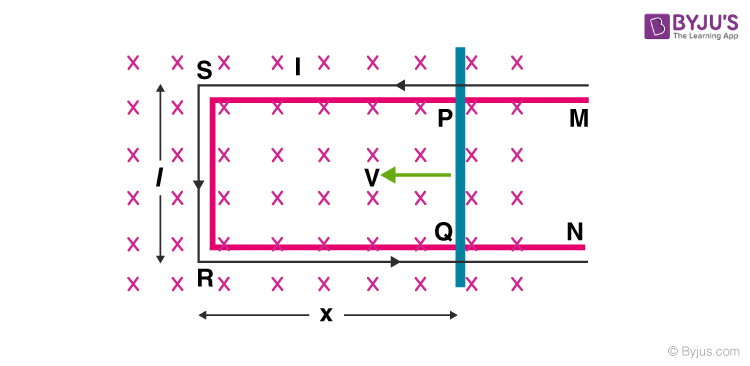# Motional emf, Electromotive Force, Induced emf

We all know that when an electrical conductor is introduced into a magnetic field, due to its dynamic interaction with the magnetic field, emf is induced in it. This emf is known as induced emf. In this article, we will learn about motional emf where emf is induced in a moving electric conductor in the presence of a magnetic field.

## Proof of motional emf• Consider a straight conductor PQ as shown in the figure, moving in the rectangular loop PQRS in a uniform and time-independent magnetic field B, perpendicular to the plane of the system.
• Let us suppose the motion of rod to be uniform at a constant velocity of v m/sec and the surface to be frictionless.
• Thus, the rectangle PQRS forms a closed circuit enclosing a varying area due to the motion of the rod PQ.
• The magnetic flux ΦB enclosed by the loop PQRS can be given as

ΦB = Blx

Where, RQ = x and RS = l, Since the conductor is moving, x is changing with time. Thus, the rate of change of flux ΦB will induce an emf, which is given by:

 $\epsilon =\frac{-d\phi _{B}}{dt}=\frac{-d}{dt}(Blx)$$=-Bl\frac{dx}{dt}=Blv$

Where, the speed of conductor (PQ), v = -dx/dt and is the formula of induced emf. This induced emf due to the motion of an electric conductor in the presence of the magnetic field is called motional emf. Thus, emf can be induced in two major ways:

• Due to the motion of a conductor in the presence of a magnetic field.
• Due to the change in the magnetic flux enclosed by the circuit.

Following is the table of links related to EMF:

This concept of motional emf can be explained with the help of the concept of Lorentz force acting on free charge carriers of the conductor. Let us consider any arbitrary charge q in the conductor PQ. As the rod moves with a constant speed v, the charge is also moving with a speed v in the presence of magnetic field B. The Lorentz force on this charge is given by:
F = qvB

The work done in moving the charge from P to Q can be given by,
W = QBvl

Since, emf is defined as the work done per unit charge,
∈ = wq=Bvl